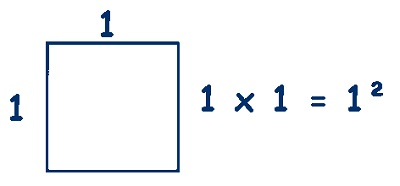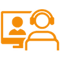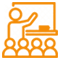# Square NumberThe number we get after multiplying an integer (not a fraction) by itself. Example: 4 ? 4 = 16, so 16 is a square number. Here are the first few square numbers: 0 (=0?0) 1 (=1?1) 4 (=2?2) 9 (=3?3) 16 (=4?4) 25 (=5?5) ?

A
B
C
D
E
F
G
H
I
J
K
L
M
N
O
P
Q
R
S
T
U
V
W
X
Y
Z

## Explore

#### SchoolPlus Program

Yearlong program for Olympiads preparation & to build necessary skills for future.

Explore MoreExplore MoreExplore MoreAssess your performance by taking topic-wise and full length mock tests.

Explore MoreExplore MoreExplore More